# Cylindrical equal-area projection

Cylindrical equal-area projection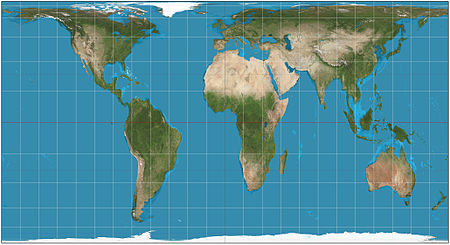Cylindrical equal-area projection of the world; standard parallel at 40°N.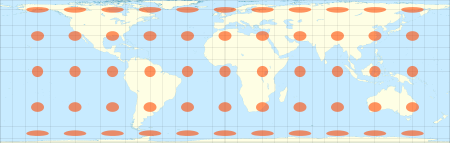The Lambert cylindrical equal-area projection with Tissot's indicatrix of deformation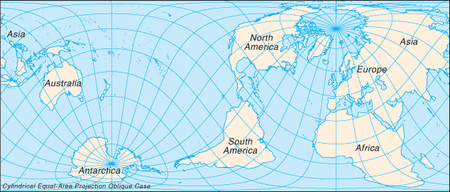Cylindrical equal-area projection with oblique orientation

In cartography, the cylindrical equal-area projection is a family of cylindrical, equal area map projections.

## Cylindrical projections

The term "normal cylindrical projection" is used to refer to any projection in which meridians are mapped to equally spaced vertical lines and circles of latitude (parallels) are mapped to horizontal lines (or, mutatis mutandis, more generally, radial lines from a fixed point are mapped to equally spaced parallel lines and concentric circles around it are mapped to perpendicular lines).

The mapping of meridians to vertical lines can be visualized by imagining a cylinder (of which the axis coincides with the Earth's axis of rotation) wrapped around the Earth and then projecting onto the cylinder, and subsequently unfolding the cylinder.

By the geometry of their construction, cylindrical projections stretch distances east-west. The amount of stretch is the same at any chosen latitude on all cylindrical projections, and is given by the secant of the latitude as a multiple of the equator's scale. The various cylindrical projections are distinguished from each other solely by their north-south stretching (where latitude is given by φ):

The only cylindrical projections that preserve area have a

• North-south compression precisely the reciprocal of east-west stretching (cosine φ): equal-area cylindrical (with many named specializations such as Gall–Peters or Gall orthographic, Behrmann, and Lambert cylindrical equal-area). This divides north-south distances by a factor equal to the secant of the latitude, preserving area but heavily distorting shapes.

Any particular cylindrical equal-area map has a pair of identical latitudes of opposite sign (or else the equator) at which the east-west scale matches the north-south-scale.

## Description

### Formulae

All cylindrical equal-area projections use the formula:$x = ( \lambda - \lambda_0 ) \cos \phi_0\,$$y = \sin \phi / \cos \phi_0\,$

where$\lambda\,$ is the longitude,$\lambda_0\,$ is the central meridian,$\phi\,$ is the latitude, and$\phi_0\,$ is the standard latitude, all expressed in radians.

Some cartographers prefer to work in degrees, rather than radians, and use the equivalent formula:$x = ( \lambda - \lambda_0 ) (\cos \phi_0) \pi / 180^\circ$$y = \sin \phi / \cos \phi_0\,$

### Simplified formula

Stripping out unit conversion and uniform scaling, the formulae may be written:$x = ( \lambda - \lambda_0 ) S\,$$y = \sin\phi\,$

Hence the sphere is mapped onto a stretched vertical cylinder. The stretch factor S is what distinguishes the variations of cylindric equal-area projection.

### Discussion

The various specializations of the cylindric equal-area projection differ only in the ratio of the vertical to horizontal axis. This ratio determines the standard parallel of the projection, which is the parallel at which there is no distortion and along which distances match the stated scale. There are always two standard parallels on the cylindric equal-area projection, each at the same distance north and south of the equator. The standard parallels of the Gall–Peters are 45° N and 45° S. Several other specializations of the equal-area cylindric have been described, promoted, or otherwise named.

Named specializations of the cylindrical equal-area projection
Projection Image Creator
(year)
Standard parallels N/S Width/height aspect ratio Notes
cylindrical equal-area$\phi_0\,$$\pi(\cos \phi_0)^2\,$ Base projection for all the others.
Lambert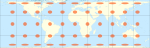Johann Heinrich Lambert
(1772)
Equator (0°)$\pi \approx 3.14$
Behrmann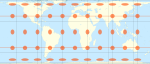Walter Behrmann
(1910)
30°$3\pi/4 \approx 2.36 \approx$ δS
Smyth equal-surface
= Craster rectangular
C. Piazzi Smyth
(1870)
37°04' 2.0
Trystan Edwards Trystan Edwards (1953) 37°24'$\approx 2.0$
Hobo–Dyer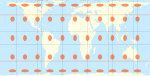Mick Dyer
(2002)
37°30'$\approx 2.0$
Gall–Peters
= Gall orthographic
= Peters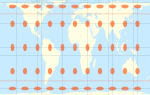James Gall
(1855)
45°$\pi/2 \approx 1.6 \approx$ Φ Promoted by Arno Peters as a new invention in 1967.
Balthasart M. Balthasart
(1935)
50°$\approx 1.3$

## History

The invention of the Lambert cylindrical equal-area projection is attributed to the Alsatian mathematician Johann Heinrich Lambert in 1772.

Arno Peters devised an equal-area map in 1967 and presented it as a "new invention in 1973."

The Tobler hyperelliptical projection, first described by Tobler in 1973, is a further generalization of the cylindrical equal-area family.

The HEALPix projection is an equal-area hybrid combination of: the Lambert cylindrical equal-area projection, for the equatorial regions of the sphere; and an interrupted Collignon projection, for the polar regions.

Wikimedia Foundation. 2010.

### Look at other dictionaries:

• Lambert cylindrical equal-area projection — In cartography, the Lambert cylindrical equal area projection, Lambert cylindrical projection, or cylindrical equal area projection is a cylindrical, equal area map projection. The invention of this projection is attributed to the Alsatian… …   Wikipedia

• cylindrical projections/cylindrical equal-area map projection — A cylindrical map projection onto a cylinder tangent to a sphere, showing the geographic meridians as a family of equal spaced parallel straight lines perpendicular to a second family of parallel straight lines. They represent the geographic… …   Aviation dictionary

• Lambert azimuthal equal-area projection — The Lambert azimuthal equal area projection is a particular mapping from a sphere to a disk. It accurately represents area in all regions of the sphere, but it does not accurately represent angles. Intuitively, it gives a planar picture of the… …   Wikipedia

• Mercator projection — of the world between 82°S and 82°N. Mercator world …   Wikipedia

• Tobler hyperelliptical projection — The Tobler hyperelliptical projection is a family of pseudocylindrical projections used for mapping the earth.It is named for Waldo R. Tobler, its inventor. It is an equal area projection. In the normal aspect, the parallels of latitude are… …   Wikipedia

• Orthographic projection (cartography) — Orthographic projection (equatorial aspect) of eastern hemisphere 30W–150E An orthographic projection is a map projection of cartography. Like the stereographic projection and gnomonic projection, orthographic projection is a perspective (or… …   Wikipedia

• Map projection — A medieval depiction of the Ecumene (1482, Johannes Schnitzer, engraver), constructed after the coordinates in Ptolemy s Geography and using his second map projection A map projection is any method of representing the surface of a sphere or other …   Wikipedia

• Stereographic projection — In geometry, the stereographic projection is a particular mapping (function) that projects a sphere onto a plane. The projection is defined on the entire sphere, except at one point mdash; the projection point. Where it is defined, the mapping is …   Wikipedia

• Gall-Peters projection — The Gall Peters projection is one specialization of a configurable equal area map projection known as the equal area cylindric or cylindric equal area projection. The Gall Peters achieved considerable notoriety in the late 20th century as the… …   Wikipedia

• Equirectangular projection — The equirectangular projection (also called the equidistant cylindrical projection, geographic projection, plate carré or carte parallelogrammatique projection or CPP) is a very simple map projection attributed to Marinus of Tyre, who Ptolemy… …   Wikipedia